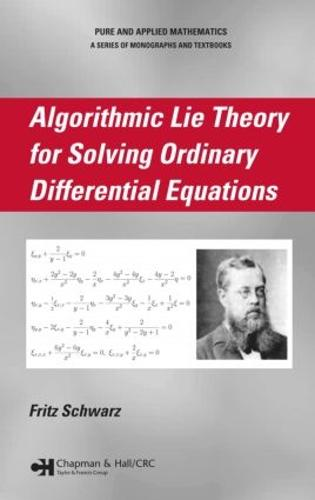•Algorithmic Lie Theory for Solving Ordinary Differential Equations - Chapman & Hall/CRC Pure and Applied Mathematics (Hardback)

(author)
£96.99
Hardback 448 Pages / Published: 02/10/2007
• We can order this

Usually dispatched within 3 weeks

Despite the fact that Sophus Lie's theory was virtually the only systematic method for solving nonlinear ordinary differential equations (ODEs), it was rarely used for practical problems because of the massive amount of calculations involved. But with the advent of computer algebra programs, it became possible to apply Lie theory to concrete problems. Taking this approach, Algorithmic Lie Theory for Solving Ordinary Differential Equations serves as a valuable introduction for solving differential equations using Lie's theory and related results.

After an introductory chapter, the book provides the mathematical foundation of linear differential equations, covering Loewy's theory and Janet bases. The following chapters present results from the theory of continuous groups of a 2-D manifold and discuss the close relation between Lie's symmetry analysis and the equivalence problem. The core chapters of the book identify the symmetry classes to which quasilinear equations of order two or three belong and transform these equations to canonical form. The final chapters solve the canonical equations and produce the general solutions whenever possible as well as provide concluding remarks. The appendices contain solutions to selected exercises, useful formulae, properties of ideals of monomials, Loewy decompositions, symmetries for equations from Kamke's collection, and a brief description of the software system ALLTYPES for solving concrete algebraic problems.

Publisher: Taylor & Francis Ltd
ISBN: 9781584888895
Number of pages: 448
Weight: 748 g
Dimensions: 235 x 159 x 25 mm